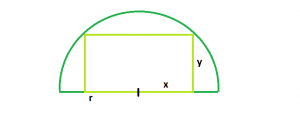# Largest rectangle that can be inscribed in a semicircle

Given a semicircle of radius r, we have to find the largest rectangle that can be inscribed in the semicircle, with base lying on the diameter.

Examples:

```Input : r = 4
Output : 16

Input : r = 5
Output :25
```

## Recommended: Please try your approach on {IDE} first, before moving on to the solution.## Recommended: Please try your approach on {IDE} first, before moving on to the solution.

Let r be the radius of the semicircle, x one half of the base of the rectangle, and y the height of the rectangle. We want to maximize the area, A = 2xy.
So from the diagram we have,
y = √(r^2 – x^2)
So, A = 2*x*(√(r^2 – x^2)), or dA/dx = 2*√(r^2 – x^2) -2*x^2/√(r^2 – x^2)
Setting this derivative equal to 0 and solving for x,
dA/dx = 0
or, 2*√(r^2 – x^2) – 2*x^2/√(r^2 – x^2) = 0
2r^2 – 4x^2 = 0
x = r/√2

This is the maximum of the area as,
dA/dx > 0 when x > r/√2
and, dA/dx < 0 when x > r/√2

Since y =√(r^2 – x^2) we then have

y = r/√2

Thus, the base of the rectangle has length = r/√2 and its height has length √2*r/2.
So, Area, A=r^2

## C++

 `// C++ Program to find the ` `// the biggest rectangle ` `// which can be inscribed ` `// within the semicircle ` `#include ` `using` `namespace` `std; ` ` `  `// Function to find the area ` `// of the biggest rectangle ` `float` `rectanglearea(``float` `r) ` `{ ` ` `  `    ``// the radius cannot be negative ` `    ``if` `(r < 0) ` `        ``return` `-1; ` ` `  `    ``// area of the rectangle ` `    ``float` `a = r * r; ` ` `  `    ``return` `a; ` `} ` ` `  `// Driver code ` `int` `main() ` `{ ` `    ``float` `r = 5; ` `    ``cout << rectanglearea(r) << endl; ` `    ``return` `0; ` `} `

## Java

 `// Java Program to find the ` `// the biggest rectangle ` `// which can be inscribed ` `// within the semicircle ` `class` `GFG  ` `{ ` ` `  `// Function to find the area ` `// of the biggest rectangle ` `static` `float` `rectanglearea(``float` `r) ` `{ ` ` `  `// the radius cannot be negative ` `if` `(r < ``0``) ` `    ``return` `-``1``; ` ` `  `// area of the rectangle ` `float` `a = r * r; ` ` `  `return` `a; ` `} ` ` `  `// Driver code ` `public` `static` `void` `main(String[] args) ` `{ ` `    ``float` `r = ``5``; ` `    ``System.out.println((``int``)rectanglearea(r)); ` `} ` `} ` ` `  `// This code is contributed ` `// by ChitraNayal `

## Python 3

 `# Python 3 Program to find the  ` `# the biggest rectangle  ` `# which can be inscribed  ` `# within the semicircle  ` ` `  `# Function to find the area  ` `# of the biggest rectangle  ` `def` `rectanglearea(r) : ` ` `  `    ``# the radius cannot ` `    ``# be negative  ` `    ``if` `r < ``0` `: ` `        ``return` `-``1` ` `  `    ``# area of the rectangle  ` `    ``a ``=` `r ``*` `r ` ` `  `    ``return` `a ` ` `  `# Driver Code ` `if` `__name__ ``=``=` `"__main__"` `: ` ` `  `    ``r ``=` `5` ` `  `    ``# function calling ` `    ``print``(rectanglearea(r)) ` ` `  `# This code is contributed  ` `# by ANKITRAI1 `

## C#

 `// C# Program to find the ` `// the biggest rectangle ` `// which can be inscribed ` `// within the semicircle ` `using` `System; ` ` `  `class` `GFG  ` `{ ` ` `  `// Function to find the area ` `// of the biggest rectangle ` `static` `float` `rectanglearea(``float` `r) ` `{ ` ` `  `// the radius cannot be negative ` `if` `(r < 0) ` `    ``return` `-1; ` ` `  `// area of the rectangle ` `float` `a = r * r; ` ` `  `return` `a; ` `} ` ` `  `// Driver code ` `public` `static` `void` `Main()  ` `{ ` `    ``float` `r = 5; ` `    ``Console.Write((``int``)rectanglearea(r)); ` `} ` `} ` ` `  `// This code is contributed ` `// by ChitraNayal `

## PHP

 ` `

OUTPUT :

```25
```

Attention reader! Don’t stop learning now. Get hold of all the important DSA concepts with the DSA Self Paced Course at a student-friendly price and become industry ready.

My Personal Notes arrow_drop_upCheck out this Author's contributed articles.

If you like GeeksforGeeks and would like to contribute, you can also write an article using contribute.geeksforgeeks.org or mail your article to contribute@geeksforgeeks.org. See your article appearing on the GeeksforGeeks main page and help other Geeks.

Please Improve this article if you find anything incorrect by clicking on the "Improve Article" button below.

Improved By : AnkitRai01, chitranayal

Article Tags :
Practice Tags :

1

Please write to us at contribute@geeksforgeeks.org to report any issue with the above content.